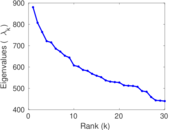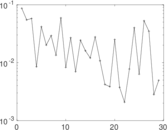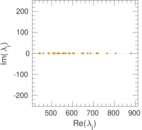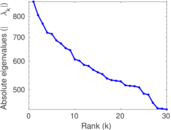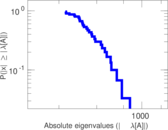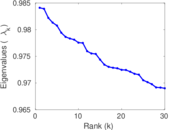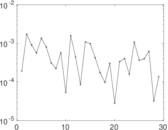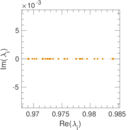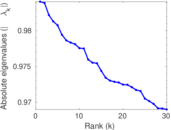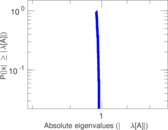This network consists of the wikilinks of the Wikipedia in the Catalan language (ca). Nodes are Wikipedia articles, and directed edges are wikilinks, i.e., hyperlinks within one wiki. In the wiki source, these are indicated with [[double brackets]]. Only pages in the article namespace are included.

 Code `Wca` Internal name `wikipedia_link_ca` Name Wikipedia links (ca) Data source http://dumps.wikimedia.org/ AvailabilityDataset is available for download Consistency checkDataset passed all tests Category Hyperlink network Node meaning Article Edge meaning Wikilink Network format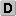Unipartite, directed Edge typeUnweighted, no multiple edges ReciprocalContains reciprocal edges Directed cyclesContains directed cycles Loops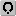Contains loops

## Statistics

 Size n = 926,636 Volume m = 38,966,215 Loop count l = 1,120 Wedge count s = 64,211,956,188 Claw count z = 883,462,519,232,847 Cross count x = 1.380 09 × 1019 Triangle count t = 2,001,725,462 Maximum degree dmax = 96,201 Maximum outdegree d+max = 4,007 Maximum indegree d−max = 96,191 Average degree d = 84.102 5 Fill p = 4.538 06 × 10−5 Size of LCC N = 926,588 Size of LSCC Ns = 698,647 Relative size of LSCC Nrs = 0.753 961 Diameter δ = 10 50-Percentile effective diameter δ0.5 = 3.152 90 90-Percentile effective diameter δ0.9 = 4.089 02 Median distance δM = 4 Mean distance δm = 3.633 54 Balanced inequality ratio P = 0.162 138 Outdegree balanced inequality ratio P+ = 0.188 326 Indegree balanced inequality ratio P− = 0.136 881 Power law exponent γ = 1.392 62 Degree assortativity ρ = −0.052 532 9 Degree assortativity p-value pρ = 0.000 00 Clustering coefficient c = 0.093 521 2 Directed clustering coefficient c± = 0.830 803 Operator 2-norm ν = 903.494 Cyclic eigenvalue π = 880.032 Reciprocity y = 0.607 344 Non-bipartivity bA = 0.520 060 Normalized non-bipartivity bN = 0.071 917 2

## Plots

### Degree distribution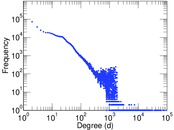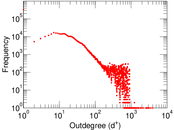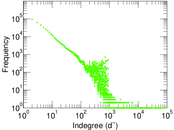### Cumulative degree distribution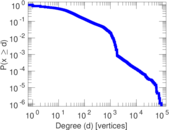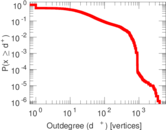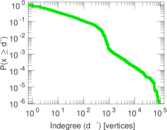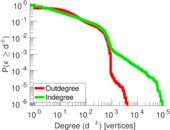### Degree assortativity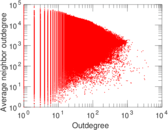### Hop distribution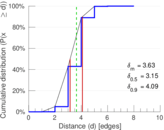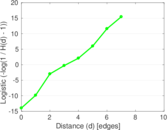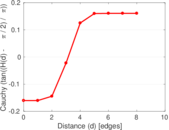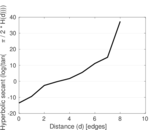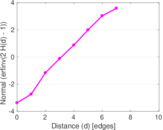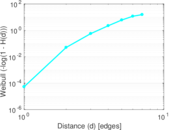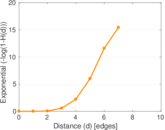### In/outdegree scatter plot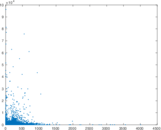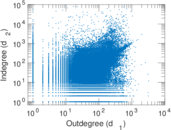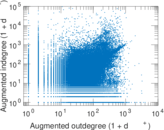### Matrix decompositions plots